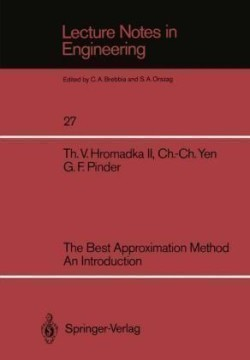# The Best Approximation Method An Introduction# The Best Approximation Method An Introduction

Na objednávku
Předpokládané dodání v pátek, 22. ledna 2021
3.435 Kč s DPH
Ušetříte 382 Kč 3.817 Kč (-10%)
The most commonly used numerical techniques in solving engineering and mathematical models are the Finite Element, Finite Difference, and Boundary Element Methods. As computer capabilities continue to impro':e in speed, memory size and access speed, and lower costs, the use of more accurate but computationally expensive numerical techniques will become attractive to the practicing engineer. This book presents an introduction to a new approximation method based on a generalized Fourier series expansion of a linear operator equation. Because many engineering problems such as the multi dimensional Laplace and Poisson equations, the diffusion equation, and many integral equations are linear operator equations, this new approximation technique will be of interest to practicing engineers. Because a generalized Fourier series is used to develop the approxi mator, a "best approximation" is achieved in the "least-squares" sense; hence the name, the Best Approximation Method. This book guides the reader through several mathematics topics which are pertinent to the development of the theory employed by the Best Approximation Method. Working spaces such as metric spaces and Banach spaces are explained in readable terms. Integration theory in the Lebesque sense is covered carefully. Because the generalized Fourier series utilizes Lebesque integration concepts, the integra tion theory is covered through the topic of converging sequences of functions with respect to measure, in the mean (Lp), almost uniformly IV and almost everywhere. Generalized Fourier theory and linear operator theory are treated in Chapters 3 and 4.
EAN
9783540175728
ISBN
3540175725
Typ produktu
Paperback / softback
Vydavatel
Springer, Berlin
Stránky
172
Jazyk
Angličtina
Rozměry
242 x 165 x 7
Sekce
Professional & Scholarly
Autoři
Hromadka, Theodore V.; Pinder, George F.; Yen, Chung-Cheng
Ilustrace
XIII, 168 pp.
Série
Lecture Notes in Engineering
Související produkty
14 299 039
knih
2 833 679
e-knih
Knižních titulů v nabídce
Osobní odběr zdarma na 10 místech po celé České republice
Největší knižní nabídka na českém internetu
Dárkové balení knih zdarma
Knihy z celého světa

### AkceptujemeTento web používá k poskytování služeb, personalizaci reklam a analýze návštěvnosti soubory cookies. Používáním tohoto webu s tím souhlasíte. Více informací
Rozumím